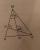# Cablecar

Funicular on Petrin (Prague) was 408 meters long and overcomes the difference 106 meters in altitude. Calculate the angle of climb.

Result

x =  15.058 °

#### Solution:Leave us a comment of example and its solution (i.e. if it is still somewhat unclear...):Be the first to comment!## Next similar examples:

1. Cable car 2Cable car rises at an angle 41° and connects the upper and lower station with an altitude difference of 1175 m. How long is the track of cable car?
2. Cable carCable car rises at an angle 45° and connects the upper and lower station with an altitude difference of 744 m. How long is "endless" tow rope?The double ladder is 8.5m long. It is built so that its lower ends are 3.5 meters apart. How high does the upper end of the ladder reach?The double ladder shoulders should be 3 meters long. What height will the upper top of the ladder reach if the lower ends are 1.8 meters apart?What is the slope of a ladder 6.2 m long and 5.12 m in height.
6. Isosceles triangleWhat are the angles of an isosceles triangle ABC if its base is long a=5 m and has an arm b=4 m.
7. 30-60-90The longer leg of a 30°-60°-90° triangle measures 5. What is the length of the shorter leg?
8. Center traverseIt is true that the middle traverse bisects the triangle?
9. Height 2Calculate the height of the equilateral triangle with side 38.
10. Right triangleLadder 16 feet reaches up 14 feet on a house wall. The 90-degree angle at the base of the house and wall. What are the other two angles or the length of the leg of the yard?
11. ClimbFor horizontal distance 4.2 km road rise by 6.3 m. Calculate the road pitch in ‰ (permille, parts per thousand).
12. An angleAn angle x is opposite side AB which is 10, and side AC is 15 which is hypotenuse side in triangle ABC. Calculate angle x.
13. LiftThe largest angle at which the lift rises is 16°31'. Give climb angle in permille.
14. AnglesThe outer angle of the triangle ABC at the vertex A is 114°12'. The outer angle at the vertex B is 139°18'. What size is the internal angle at the vertex C?
15. Obtuse angleThe line OH is the height of the triangle DOM, line MN is the bisector of angle DMO. obtuse angle between the lines MN and OH is four times larger than the angle DMN. What size is the angle DMO? (see attached image)
16. Find the 9Find the missing angle in the triangle and then name triangle. Angles are: 95, 2x+15, x+3
17. SinesIn ▵ ABC, if sin(α)=0.5 and sin(β)=0.6 calculate sin(γ)